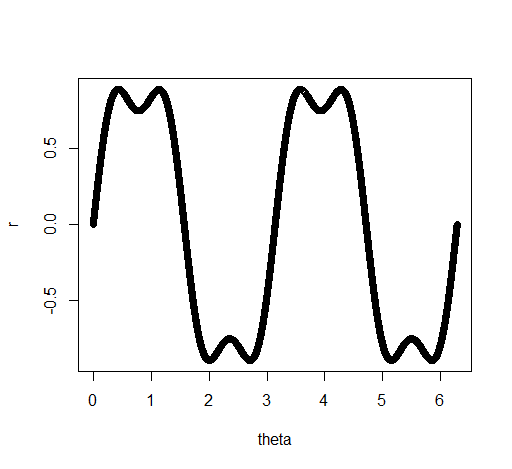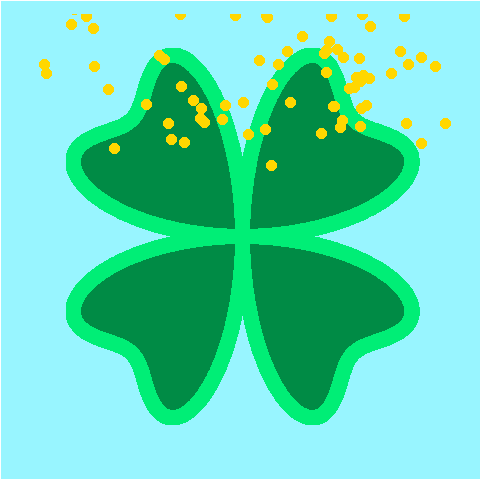Posted by Natalie Patten on 3/16/15 10:56 AMFind me on:

--------------------------------------------------------Happy St. Patrick’s Day! We continue our holiday blog series with a statistical graph of a shamrock that was made in R using the ggplot2 package. ggplot2 is a useful tool in data visualization.

To make the shamrock, we must brush up on our high school trigonometry and use the mathematical formula:

r = sin2θ + 1/4sin6θ

where 0  θ ≤ 2π. We plot theta on xy coordinates:

x = r * cosθ
y = r * sinθ

This gives us the outline. Now we want to fill in the shamrock. To do this, we divide the shamrock into four quadrants. A plot of theta and r below shows what happens in the quadrants.When  θ ≤ π/2 or when π  θ ≤ 3π/2, r is positive. To fill in the shamrocks for those quadrants, we generate random points and keep them only if r falls between 0 and the outline of the shamrock.  Likewise, when π/2 ≤ θ ≤ 0 or when 3π/2  θ ≤ 2π, r is negative. For these quadrants, we want randomly generated points where r falls between the outline of the shamrock and 0.

For each point (θi , ri) that we keep, we find the x and y values using the equations above. When all four quadrants are complete, we put them into one data frame so that all the points will plot together. You can play with the size of the dots and the size of the sample that you select to eliminate white space in the shamrock.

Now, let’s make it rain gold…

To do this, we use the animation package. We generate random points for x (between -1 and 1) and y (between .5 and 1.5, so that most of the gold falls from “above” the plot). We also generate a random variable (fall.speed) between .05 and .25 that will serve as the rate that each gold coin falls.

We create a loop that creates each “frame” for our animation. In each frame, the y value of the coin decreases by fall.speed. Then, we plot it using ggplot and use the animation package to put together each individual frame into a single animation.The code is available here. Have a safe and fun St. Patrick’s Day!Topics: data analytics, R